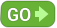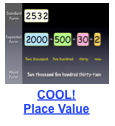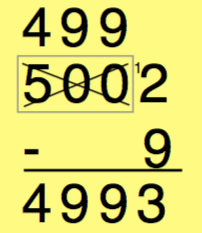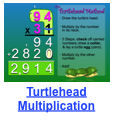##### Print or Share Flyer# Lesson Dashboard

## Multiplication/ Division

### Lesson 1: Zeroes & OnesLesson Focus:

Lesson 1 is the first and only lesson using the Rule Strategy. The Multiplication facts in this group are typically very simple for students to master. The commutative property of Multiplication is emphasized in this and all successive lessons. Once students understand the Zero and Identity properties, all Zeroes and Ones facts can be easily mastered.

### Lesson 2: Terrific TwosLesson Focus:

Lesson 2 is the first lesson in the Trick Group. Fluency with Addition Doubles facts ensures near-instant automaticity with all Multiplication facts in this group. The other “trick” presented is skip-counting by Twos. Once students understand the strategies (Double the Other Number or Count by Twos), all Twos Multiplication facts can be easily mastered.

### Lesson 3: Fantastic FivesLesson Focus:

Lesson 3 offers two “tricks” for the Fives facts: Count by Fives and Fives Product Pattern. Skip-counting is practiced and students are also taught the concept of half, a necessary foundation for utilizing the Product Pattern trick. Students can apply the trick learned in this lesson to ALL Fives facts.

### Lesson 4: Noteworthy NinesLesson Focus:

Lesson 4 uses the trick strategy. Two tricks are presented: Finger Factor and Nines Product Pattern. The focus facts above are the only remaining Nines facts for students to master. This is the last lesson to contain six focus facts. Future lessons contain only three focus facts each.

### Lesson 5: Special SixesLesson Focus:
6×4, 6×6, 6×8, Multiplication commutative counterparts (4×6, 6×6, 8×6) and Division counterparts More Info

Lesson 5 is the last lesson using the trick strategy and the first lesson to include only THREE focus facts (rather than six). The “Half-Whole Trick” taught in this lesson can be applied anytime the number 6 is multiplied by an even number. It has amazed even the most experienced educators! Once students understand the Half-Whole Trick, the products of these Sixes facts are simple for students to recall.

### Lesson 6: Fun Facts 1Lesson Focus:
7×6, 3×4, 7×8, Multiplication commutative counterparts (6×7, 4×3, 8×7) and Division counterparts More Info

Lesson 6 is the first lesson using the chant strategy. Without a strong strategy, Multiplication facts in this group are very challenging for some students to retain. However, the chants applied to each of these facts make them simple for students to recall and are among students’ favorite facts to practice!

### Lesson 7: Fun Facts 2Lesson Focus:
6×3, 7×3, 8×4, Multiplication commutative counterparts (3×6, 7×3, 8×4) and Division counterparts More Info

Lesson 7 is the second lesson using the chant strategy. Students realize that even some of the “harder” facts- 6×3, 7×3, and 8×4 are actually simple when using a strategy that makes the facts meaningful. The Lesson 7 chants are simple to remember and fun to recite. The 6×3 chant uses a familiar beat (We will, we will ROCK YOU!)

### Lesson 8: Simple Squares

Lesson 8 is the last lesson using the chant strategy. As with Lessons 5-7, there are only three focus facts in this group, so students are never intimidated or overwhelmed. Students enjoy the Lesson 8 chants. Students have no trouble remembering these chants, leading to rapid recall. All remaining “Squares” facts have been or will be covered in other lessons.

### Lesson 9: Final FactsLesson Focus:
8×3, 4×7, 4×4, Multiplication commutative counterparts (3×8, 7×4, 4×4) and Division counterparts More Info

The Lesson 9 focus facts make up the last of the single-digit Multiplication facts. Once students pass this lesson, mastery of all Multiplication facts has been achieved. Use the Factivation!® Post-Assessment Tool after this lesson.

#### GETTING STARTED:## More Factivation!® Math Resources

### Place ValueTeach place value to the thousands using our Teacher Tube top-rated COOL! Place Value video.

### Subtraction StrategySuper Simple Subtraction across Zeroes!  This quick method deepens understanding of place value.

### 2 by 2 MultiplicationThe Turtlehead Method is a fun and quirky video that simplifies the steps of the traditional algorithm.  Be sure to read the usage instructions!